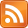The complex number $z = a + bi$ can be drawn. Plot the imaginary part on the $y$-axis, and the real part on the $x$-axis.This lets us see complex numbers as arrows. So a complex number has a certain length and forms a certain angle with the $x$-axis. (It's the same idea as polar form in the ordinary $xy$-plane).

When you add another complex number $z'$ to $z$, you get a third arrow which has the sum of the $x$-coordinates and the sum of the $y$-coordinates. When you multiply $z$ by $z'$, you get a third arrow which has the product of $z$ and $z'$'s lengths and the sum of $z$ and $z'$ angles.

Why is the second fact true? Here's one way you might discover it, assuming you were familiar with rotation matrices. Let's multiply $a + bi$ and $c + di$ algebraically:

$$\begin{matrix} (a + bi)(c + di) & = & ac + adi + bci + bdi^2\\ & = & ac + adi + bci - bd\\ & = & (ac - bd) + (ad + bc)i\\ \end{matrix}$$

Now let's see $a + bi$ as a vector and think of multiplication of $a + bi$ by $c + di$ as instead being action (left-multiplication) by some matrix on that vector.

$$\begin{bmatrix}? & ?\\? & ?\end{bmatrix}\begin{bmatrix}a\\b\end{bmatrix} = \begin{bmatrix}ac - bd\\ad + bc\end{bmatrix}$$

You can see what this matrix must be: it's

$$\begin{bmatrix}c & -d\\d & c\end{bmatrix}\begin{bmatrix}a\\b\end{bmatrix} = \begin{bmatrix}ac - bd\\ad + bc\end{bmatrix}$$

Now think about $c + di$ as something with an angle $\theta$ and a length $r$ and write it as $c + di = r\cos(\theta) + ir\sin(\theta)$. Substitute in and you get:

$$\begin{matrix} \begin{bmatrix}c & -d\\d & c\end{bmatrix} & = & \begin{bmatrix}r\cos(\theta) & -r\sin(\theta)\\ r\sin(\theta) & r\cos(\theta)\end{bmatrix}\\ & = & r\begin{bmatrix}\cos(\theta) & -\sin(\theta)\\ \sin(\theta) & \cos(\theta)\end{bmatrix} \end{matrix}$$

So the mysterious matrix, which performs multiplication by $c + di$, has turned out to be the rotation matrix for $\theta$, times a scalar.

$$(c + di)(a + bi) = r\begin{bmatrix}\cos(\theta) & -\sin(\theta)\\ \sin(\theta) & \cos(\theta)\end{bmatrix}\begin{bmatrix}a\\b\end{bmatrix}$$

The rotation matrix says to add the angles, because that's what rotation does. The scalar $r$ says to multiply the lengths.